# Analyzing Profit Margins: A Guide for Investors

Discover how to find and analyze various profit margins for stock analysis, including gross, operating, EBIT, pre-tax, net, and free cash flow profit margins.”

1. Introduction to Margins

1. The Importance of Margins in Stock Analysis

2. Types of Margins

1. Gross Profit Margin

2. Operating Profit Margin

3. EBIT Profit Margin

4. Pre-tax Profit Margin

5. Net Profit Margin

6. Free Cash Flow Profit Margin

3. Formulas for Margin Analysis

1. Calculating Margins

4. Using Stratosphere.io for Margin Analysis

5. Conclusion

6. FAQs

## Introduction to Margins

### The Importance of Margins in Stock Analysis

When it comes to stock analysis, financial ratios play a crucial role in determining the health and performance of a company. One of the most significant ratios to consider is the profit margin. Profit margins provide an insight into a company's profitability, efficiency, and competitive advantage. A firm with high profit margins demonstrates effective cost control and strong market positioning, which can be a key indicator of potential success and growth.

It is important to understand margins as they flow through financial statements.

But also:

✅ Where they are today

✅And how they have changed over time as the business becomes more… or less, profitable.

For this analysis, we will use Visa (V) as it is an example of a company with EXCEPTIONAL margins that are best in class of any business, maybe ever.

## Types of Margins

There are several types of profit margins that investors should take into account when analyzing a stock. Each margin reflects different aspects of a company's financial health and performance. Let's discuss these margins in detail.

## Margins

• Measures the percentage of revenue exceeding the cost of goods sold (COGS)
• Formula: (Gross Profit / Revenue) x 100

### Gross Profit Margin

Gross profit margin is the ratio of gross profit to revenue. It represents the percentage of revenue that exceeds the cost of goods sold (COGS). A higher gross profit margin indicates that a company is efficient in managing its production costs and has a strong pricing strategy.

Gross Profit Margin = (Gross Profit / Revenue) x 100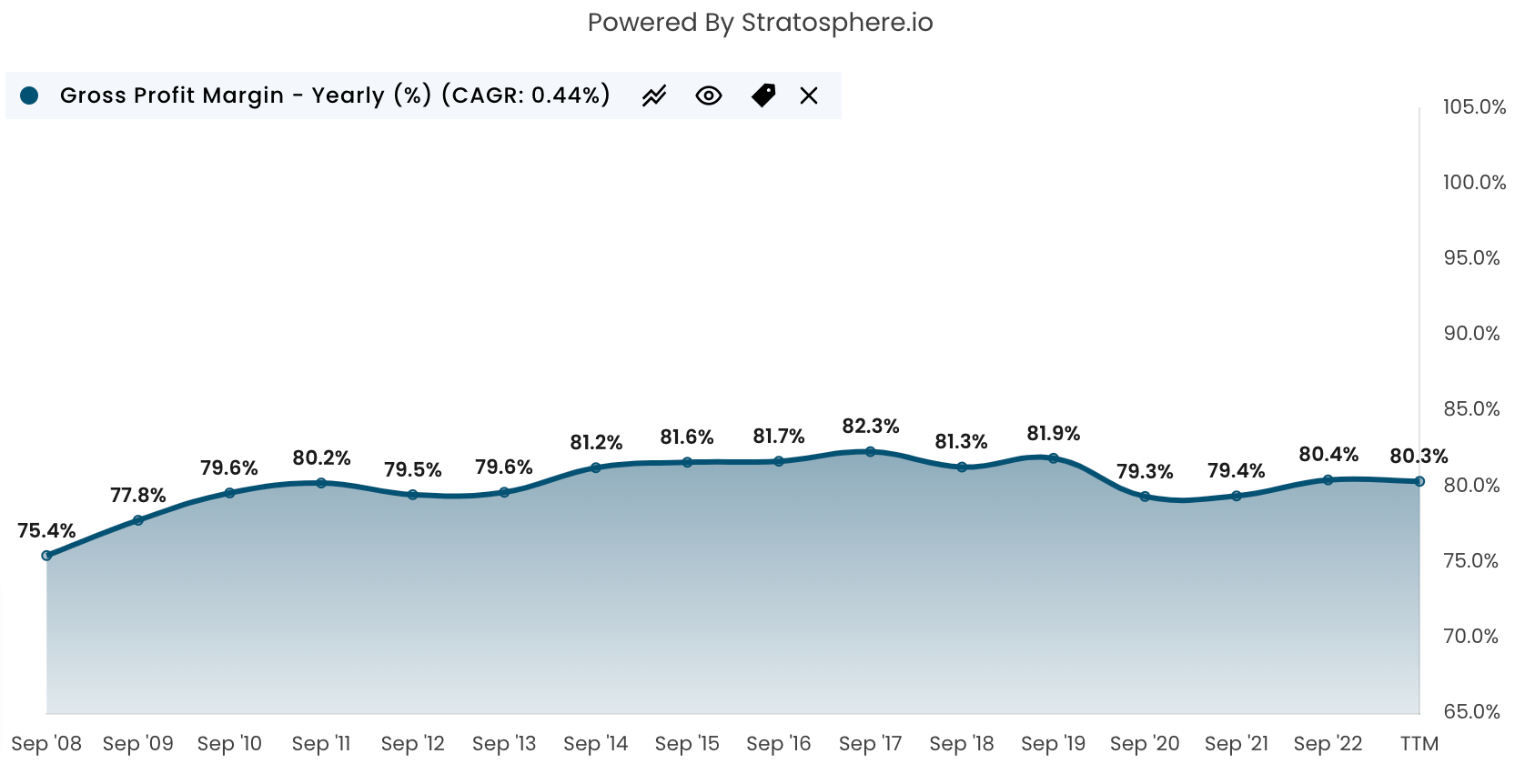### Operating Profit Margin

Operating profit margin is the ratio of operating income to revenue. It measures the percentage of revenue left after deducting the cost of goods sold and operating expenses. A higher operating profit margin demonstrates that a company can effectively manage its operating costs and has a sustainable business model.

Operating Profit Margin = (Operating Income / Revenue) x 100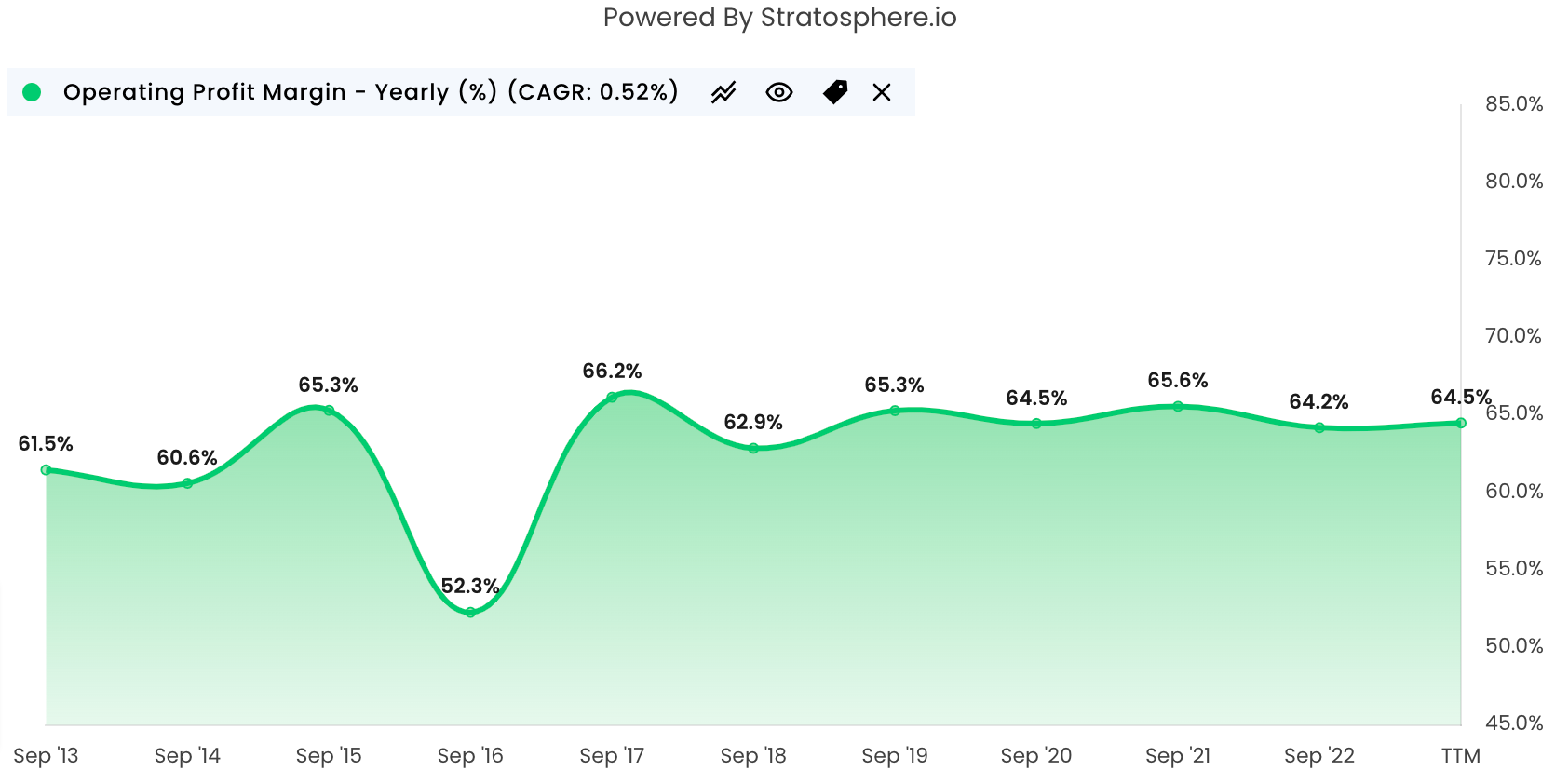### EBIT Profit Margin

EBIT (Earnings Before Interest and Taxes) profit margin is the ratio of EBIT to revenue. It reflects a company's profitability before considering the impact of interest and taxes. A higher EBIT profit margin suggests that a company has a strong operating performance and can generate significant profits.

EBIT Profit Margin = (EBIT / Revenue) x 100

### Pre-tax Profit Margin

Pre-tax profit margin is the ratio of pre-tax income to revenue. It measures the percentage of revenue that remains before accounting for income taxes. A higher pre-tax profit margin indicates that a company has a strong financial performance and can generate considerable income before taxes.

Pre-tax Profit Margin = (Pre-tax Income / Revenue) x 100

### Net Profit Margin

Net profit margin is the ratio of net income to revenue. It represents the percentage of revenue that remains after accounting for all expenses, including taxes. A higher net profit margin demonstrates that a company is efficient in managing its overall costs and can retain a significant portion of its revenue as profit.

Net Profit Margin = (Net Income / Revenue) x 100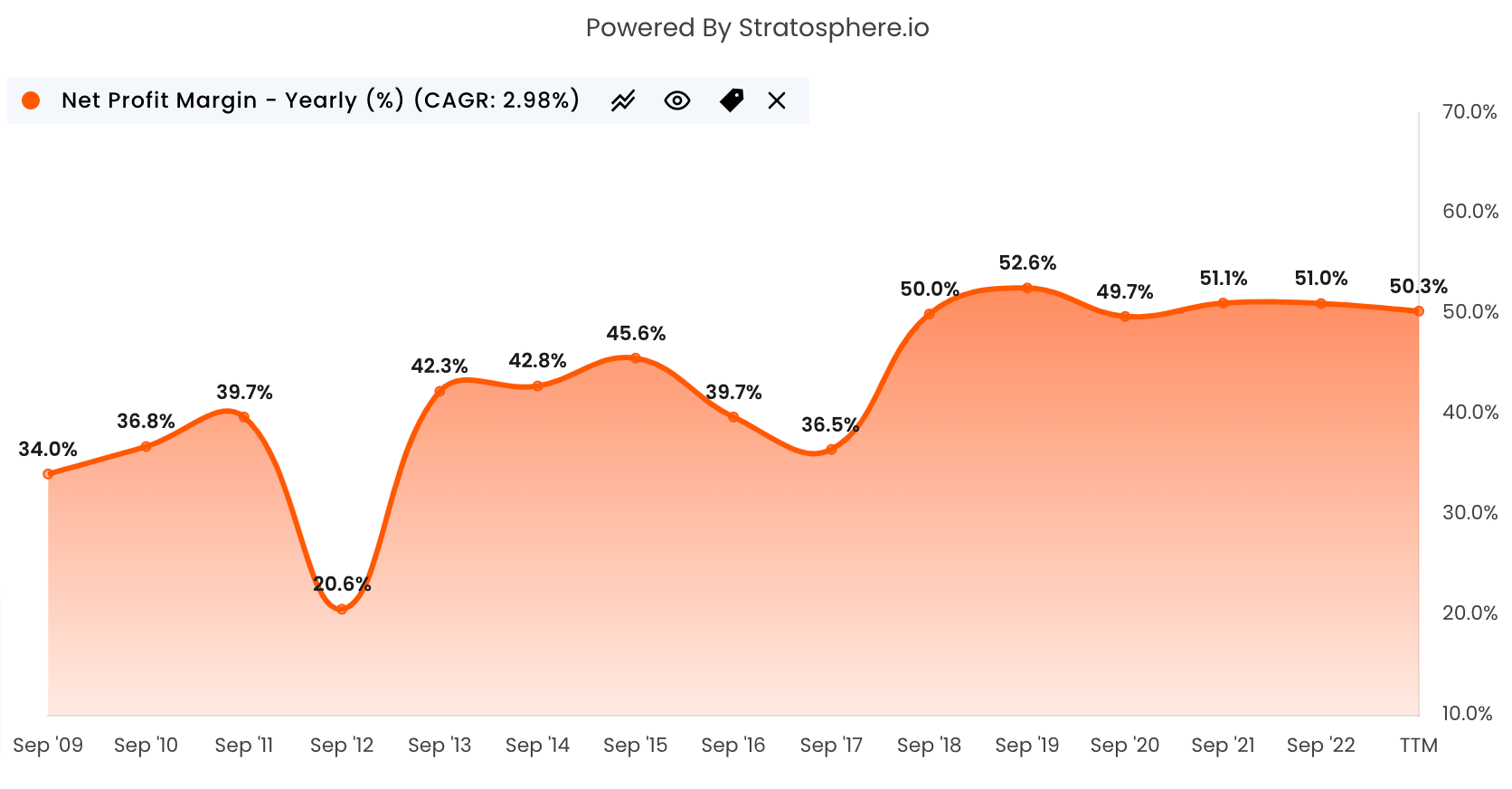### Free Cash Flow Profit Margin

Free cash flow profit margin is the

ratio of free cash flow to revenue. It represents the percentage of revenue that remains after accounting for all cash expenses, including capital expenditures and changes in working capital. A higher free cash flow profit margin indicates that a company generates a substantial amount of cash from its operations, which can be used for reinvestment, paying dividends, or reducing debt.

Free Cash Flow Profit Margin = (Free Cash Flow / Revenue) x 100

The types of metrics you will get in summary on Stratosphere.io company pages

## Formulas for Margin Analysis

### Calculating Margins

To calculate these margins for stock analysis, use the formulas provided above.

Remember to gather accurate financial data for the company you're analyzing, such as revenue, gross profit, operating income, EBIT, pre-tax income, net income, and free cash flow. These figures can be found in a company's financial statements, such as the income statement and cash flow statement.

## Using Stratosphere.io for Margin Analysis

Stratosphere.io is a valuable resource for investors who want to analyze stock margins. This platform provides all the financial data you need for various stocks, making it easy to calculate margins and evaluate a company's financial performance. Let's look at two examples from Stratosphere.io - Apple (AAPL) and Microsoft (MSFT).

### Apple vs. Microsoft Example

To analyze Apple's financial performance, visit the following link: Apple AAPL

To analyze Microsoft's financial performance, visit the following link: Microsoft MSFT

You can access the two company pages separately  which includes revenue, gross profit, operating income, EBIT, pre-tax income, net income, and free cash flow. Utilize the formulas mentioned above to calculate Microsoft's profit margins and evaluate its financial performance.

Or, you can view them side by side with our Fundamental Charting feature.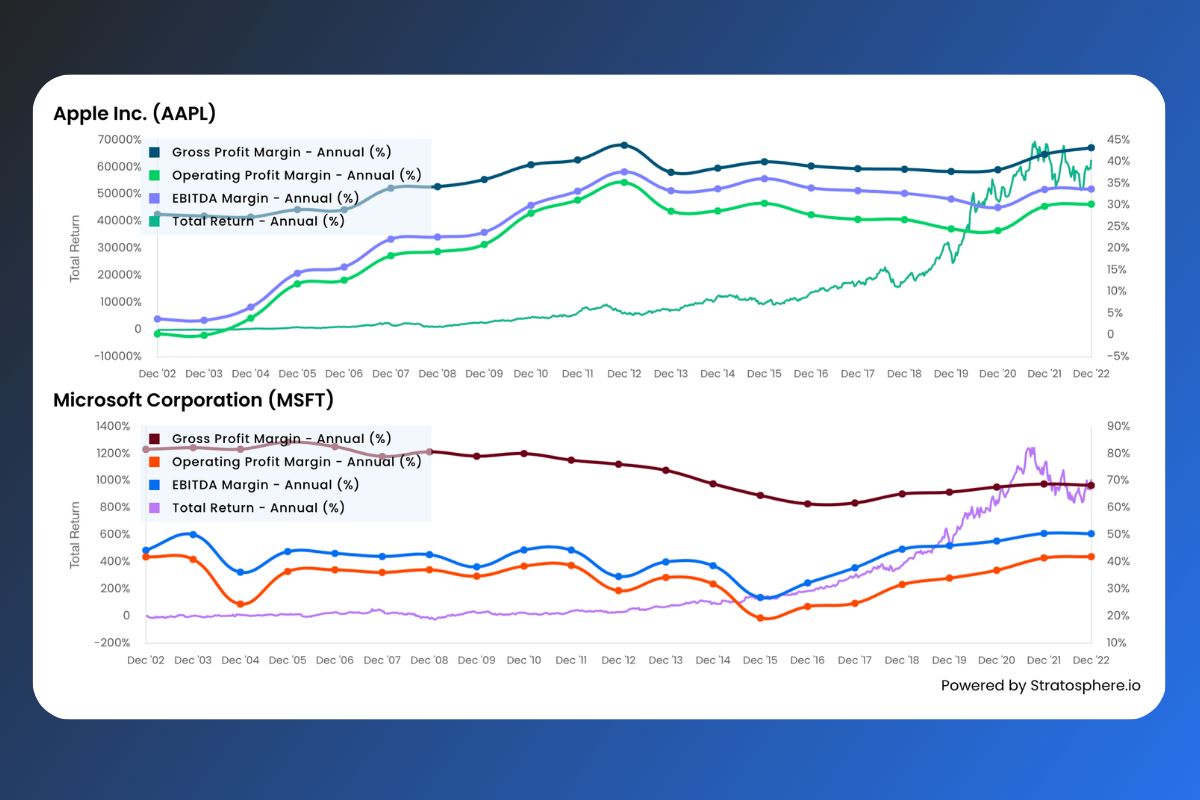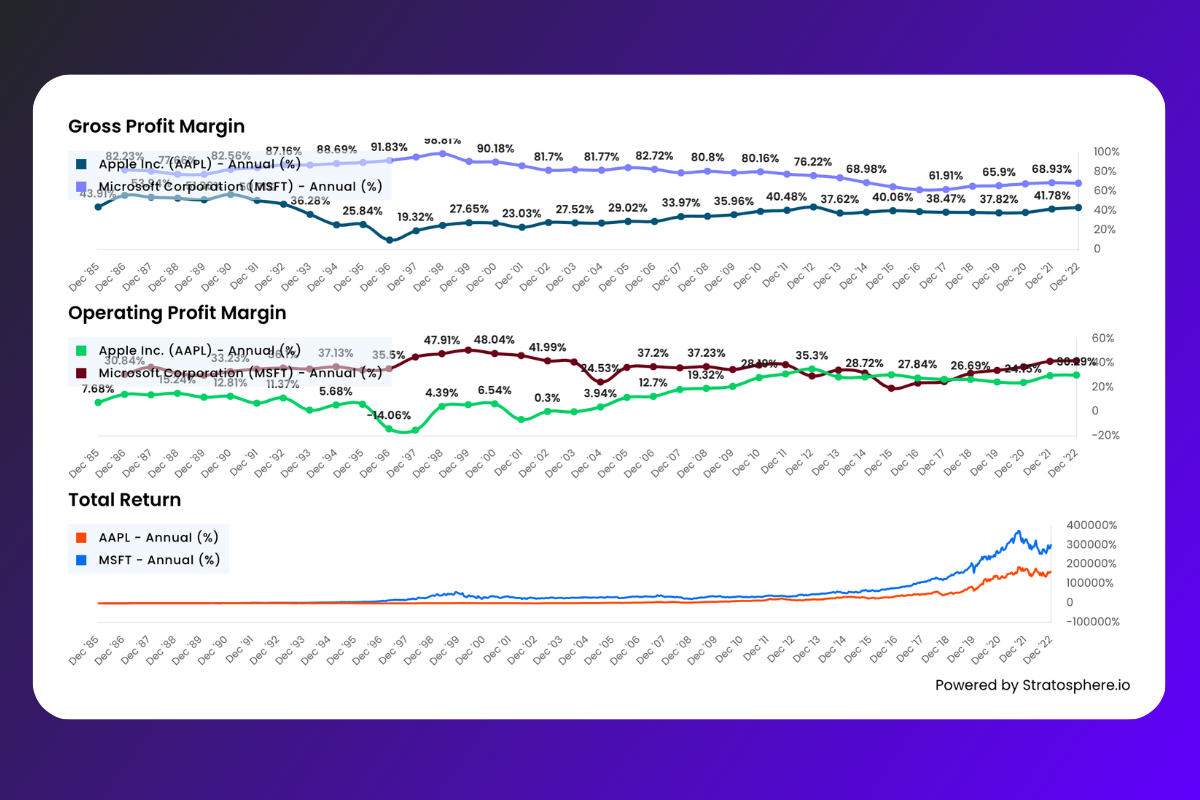## Conclusion

Analyzing profit margins is a critical aspect of stock analysis that can provide valuable insights into a company's financial health, efficiency, and competitive advantage.

By understanding and calculating various profit margins, investors can make informed decisions about which stocks to invest in. Stratosphere.io offers an excellent platform to access financial data for numerous stocks, making it easier for investors to perform margin analysis and make informed investment decisions.

FAQs

1. What is the importance of profit margins in stock analysis?

Profit margins are essential in stock analysis because they provide insights into a company's profitability, efficiency, and competitive advantage. Higher profit margins indicate effective cost control, strong market positioning, and potential for growth.

1. What are the different types of profit margins?

The different types of profit margins include gross profit margin, operating profit margin, EBIT profit margin, pre-tax profit margin, net profit margin, and free cash flow profit margin.

1. How do I calculate profit margins?

Use the formulas provided in this article to calculate profit margins, ensuring that you have accurate financial data for the company you're analyzing.

1. Where can I find financial data for various stocks?

Stratosphere.io is a valuable resource that offers financial data for numerous stocks, making it easy for investors to calculate margins and evaluate a company's financial performance.

1. How can I use Stratosphere.io to analyze profit margins for specific stocks?

Visit the Stratosphere.io page for the stock you're interested in analyzing, such as Apple or Microsoft. On the stock's page,